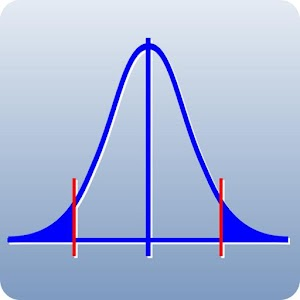# Q-DAS DistributionsThis app is intended for users requiring quick and reliable solutions to statistical questions in school, university and work using their tablet PC. For example, this app lets you calculate the probability for winning the lottery just as easily as the critical values of a hypothesis test. All the results may be displayed in graphics and charts. The app is distinguished by a user-friendly interface and reliable numerics.Distributions supported in this full version:Continuous random variables:Normal distribution, folded normal distribution, Rayleigh distribution, exponential distribution, Weibull distribution, Chi square distribution, t-distribution, range distribution (w-distribution) and F-distribution.Discrete random vairables:Hypergeometric distribution, Poisson distribution and Binomial distribution. 应用大小：:0.7197265625 MB 价格: \$ 100.41 浏览人数:1 排名: 4